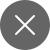# Machine Learning- Intro to Supervised Learning

2+

Supervised Machine Learning algorithms like linear regression, logistic regression, etc are said to be the starting point of the journey of a Data Scientists or a Machine Learning specialist.

From today onwards we will be starting a series of articles/tutorials based on supervised learning algorithms but before that let us take a look and get introduced to what are the use cases of supervised learning where it can prove to be beneficial, we will also give a glimpse of linear regression to start with.

Supervised Learning is a subset of Machine Learning. Machine Learning is of three types:

1. Supervised Learning
2. Unsupervised Learning
3. Reinforcement Learning

We will discuss the other two supervised learning in detail in some other articles of ours.

### What is Supervised Learning?

Supervised Learning is based on the input of the variable giving an output, but this is the same for every type of tech, every type of programming, be it web development, android development, etc, how is it different from the rest?

In supervised learning, the input variables of the dataset are trained, to train the data model, the dataset is split using the train_test_split function in Python, this function in Python helps us to split the data, it is up to us to decide as to what percentage of data we want to keep in the train and test data, usually we keep 70% data in train and 30% in the test data, it also depends on the size and type of the data set. Refer to the screenshot below to know how the train_test_split function is used.

After we have split the model we perform a moel. fit, a function to fit the model for training the data which is fitted in the trained model. Refer to the screenshot below to know more:

After we’ve have done the aforementioned procedure, the trained model is used to predict the input(test) data, that very input or labeled data is used to predict an output value of the selected or desired variable. To help better understand we will take an example of a simple use case.

### Use case: Prediction of sales

Suppose if we have data of 12 months of sales from August to June. The number of sales that happened in these 12 months will be chosen as the label data. The labeled data will be the sales data of 12 months(refer to the image below). The sales data below shows the number of sales of Volkswagen cars in a particular region.

Now, we have to predict the sales for the next few months or the next 12 months, then based on the past we will be able to predict the sales for the next year using a simple supervised learning algorithm Linear Regression. The prediction of future sales will help you to take proper business decisions, of course, certain other procedures like the sentimental analysis can also be used to understand the customer sentiment and other procedures as well to make much better and advanced business decisions, but for beginners, Linear Regression is fair enough at an academic level.

The data of sales in an example for a supervised learning model to learn because it learns by example.

We write all our articles by keeping the aspirants in mind as aspirants and data literacy is our mission and vision.

The labeled input can also be termed as a vector in this type of machine learning method.

The supervised learning method deals with both regression and classification, below are the most commonly used and prominent models used in the day to day business purposes:

1. Linear Regression
2. Logistic Regression
3. Naive Bayes
4. Support Vector Machines(SVM)
5. Decision Tree
6. Random Forest
7. K Nearest Neighbors(KNN)

Supervised Learning is of two types:

1. Regression
2. Classification

Regression– It is a mathematical equation that defines y as a function of the variables of x. In linear regression, y function defines a straight line which is equivalent to mx+c, where m is the slope and c is the intercept. In terms of machine learning, y is the continuous outcome/predictor variable, while x is multiple predictor variables. The continuous outcome predictor is based on multiple variables.

Classification– The classification technique identifies or classifies data into a set of classes. The most probable & common use cases of classification are breast cancer classification, face detection, etc. Classification is a widely used technique in healthcare, crime scene detection.

To test the accuracy of a classification model one uses a confusion matrix, to know more about what it is and how is the confusion matrix implemented click here.

That’s it for now, stay tuned and read this space regularly for more such machine learning tutorials.2+

### 5 Responses

1. October 21, 2020

[…] Supervised learning is a machine learning technique that helps a machine learn various classification and recognition […]

0
2. November 20, 2020

[…] tree algorithms are a type of supervised machine learning algorithm that can be used for both regression and classification. But is mainly considered to be used for […]

0
3. December 5, 2020

[…] forest is a supervised machine learning algorithm that can be used for solving classification and regression problems both. However, mostly it is […]

0
4. December 30, 2020

[…] behind logistic regression? If you don’t know about logistic regression, then it is a well known supervised machine learning algorithm used for classification problems. It helps in predicting a categorical dependent variable […]

0
5. January 24, 2021

[…] Supervised Learning […]

0